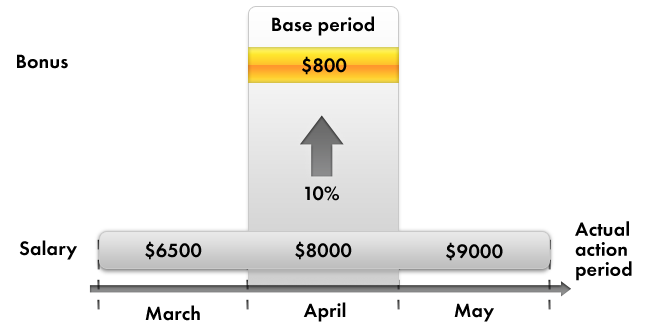# Dependency by base period

A calculation type can influence the source data of another calculation type.

As an example, consider bonuses calculated as a percentage of salary. When salary changes, a bonus requires recalculation based on the new salary amount. In other words, the modified salary amount serves as the base for bonus calculation.

Additionally, since the salary is calculated for a given period, the bonus calculation requires not just the basic salary amount, but rather the total that is calculated for the period affecting the bonus calculation. We will refer to that period as a base period, and we will refer to this dependency between calculation types as the dependency by base period.

For example, consider the accrual of bonuses for April. The bonus is calculated as 10% of the total calculated salary. So you need to analyze all the records affecting the accrual of salary for the base period you deal with, in this case for the month of April.

Suppose that the total salary is \$8 000, so the bonus would come to \$800 (fig. 17.1).Fig. 17.1. Bonus dependency on salary by base period

Next page: Displacement by action period

Be the first to know tips & tricks on business application development!